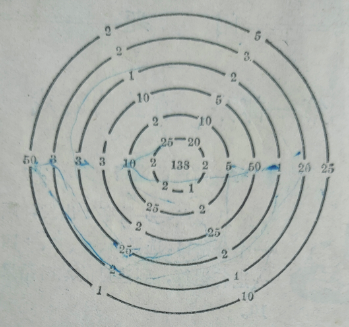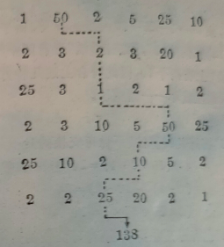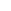# 有益的游戏-通往“数字之宫”中心的道路## 问题：通往“数字之宫”中心的道路## 答案及解题思路50+50+25+10+2+1=138

50+50+25+5+5+3=138

50+50+ 20+10+5+3=138

50+25+25+25+10+3=138

50+ 25+20+ 20+20+3=138

### 目录[+]